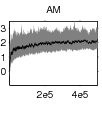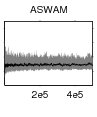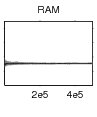Want to share your content on R-bloggers? click here if you have a blog, or here if you don't.Matti Vihola has posted a new paper on arXiv about adaptive (random walk) Metropolis-Hastings algorithms. The update in the (lower diagonal) scale matrix is$S_nS_n^text{T}=S_{n-1}left(mathbf{I}_d-eta_n[alpha_n-alpha^star]dfrac{U_nU_n^text{T}}{||U_n||^2}right)S^text{T}_{n-1}$

where

•$alpha_n$ is the current acceptance probability and$alpha^star$ the target acceptance rate;
•$U_n$ is the current random noise for the proposal,$Y_n=X_{n-1}+S_{n-1}U_n$;
•$eta_n$ is a step size sequence decaying to zero.

The spirit of the adaptation is therefore a Robbins-Monro type adaptation of the covariance matrix in the random walk, with a target acceptance rate. It follows the lines Christophe Andrieu and I had drafted in our [most famous!] unpublished paper, Controlled MCMC for optimal sampling. The current paper shows that the fixed point for$S_n$ is proportional to the scale of the target if the latter is elliptically symmetric (but does not establish a sufficient condition for convergence). It concludes with a Law of Large Numbers for the empirical average of the$f(X_n)$ under rather strong assumptions (on f, the target, and the matrices$S_n$). The simulations run on formalised examples show a clear improvement over the existing adaptive algorithms (see above) and the method is implemented within Matti Vihola’s Grapham software. I presume Matti will present this latest work during his invited talk at Adap’skiii.

Ps-Took me at least 15 minutes to spot the error in the above LaTeX formula, ending up with S^text{T}_{n−1}: Copy-pasting from the pdf file had produced an unconventional minus sign in n−1 that was impossible to spot!

Filed under: R, Statistics Tagged: acceptance rate, Adapski, adaptive MCMC methods, controlled MCMC, Grapham, LaTeX, Metropolis-Hastings